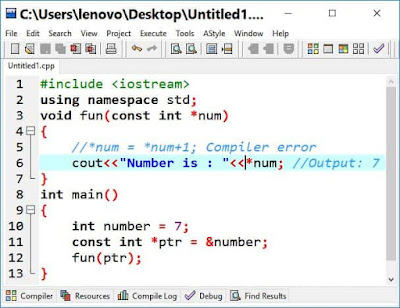## Pointers and Call by Reference

The mechanism behind calling a function is that, when we call a function we pass it some variables. The values of these variables are used by the called function. In call by value, the values of these variables are copied somewhere else in the memory. Then control goes to the called function and this copy of values is used in the function. If called function changes the value of parameter, this change is only in copies, not in original value of variable.

Now If we have to pass a huge number of values to a function, it is not suitable to copy these huge numbers of values in the memory as memory usage should be optimum. In such cases, it is better to pass the memory address (reference) of the variables, which is a call by reference phenomenon.

void fun(int *num)
{
*num = *num+1;
cout<<*num;
//Output: 8
}
int main()
{
int number = 7;
fun(&number);
cout<<number;
//Output: 8
}

The problem with call by reference is that we are letting the function to change the original values at their memory address as in above code, called function increment value by 1 and output is 8. In main function, value of variable number also becomes 8. It means that called function has control over the original value. Sometimes, we want to do this according to the requirement of the logic of the program. At some other occasion, we may pass the addresses for efficiency while not affecting the values at that addresses.

To let the called function not change the values, const keyword is used with the passing parameter.

## There are two usage of const keyword

• Constant pointer to a variable
• Pointer to a constant variable

## Constant pointer to a variable

Let’s look at the use of constant pointer. Consider the following line of declaration:

int *const myptr=&x;

Here myptr is constant pointer to integer variable x. It means that the value (memory address) of pointer myptr cannot be changed. As myptr is constant pointer so we cannot initialize it with another memory address.

Constant pointer should be initialized at the time of declaration as after declaration it cannot be re-initialized as writing below code, compiler gives error

int *const myptr;
myptr = &x;
//Compiler Error

Below is the correct way of constant pointer declaration and initialization

int *const myptr=&x;

Using constant pointer

• The value of pointer cannot be changed as

int *const myptr=&x;
myptr = &y;
//Compiler Error

• The value of variable x can be changed as

int x = 10;
int *const myptr= &x;
cout<<*myptr;
//output: 10

= 20;
cout<<*myptr;
//output:20

## Pointer to a constant variable

By changing const keyword position and taking it at the beginning, the variable x becomes constant as below

const int *myptr= &x;

Here myptr is a pointer to a constant integer variable x. Using pointer to a constant variable

•    The value of pointer can be changed as

const int *myptr= &x;
myptr = &y;
//No Error

•    Then value of variable x cannot be changed as

int x = 10;
int *const myptr= &x;
= 20;
//compiler error as
//value of variable
//cannot be changed

Pointer to a constant variable is used when we want the called function not to change of value of passing parameter.

Write a C++ program in which call a function using "Call by Reference" mechanism and restrict called function from changing value of parameter.Call by Reference in C++
OutputCall by Reference in C++ Output